## 高中数学知识点全总结：高考数学解题技巧及公式大全（2023参考）

### 一. 高中数学知识点全总结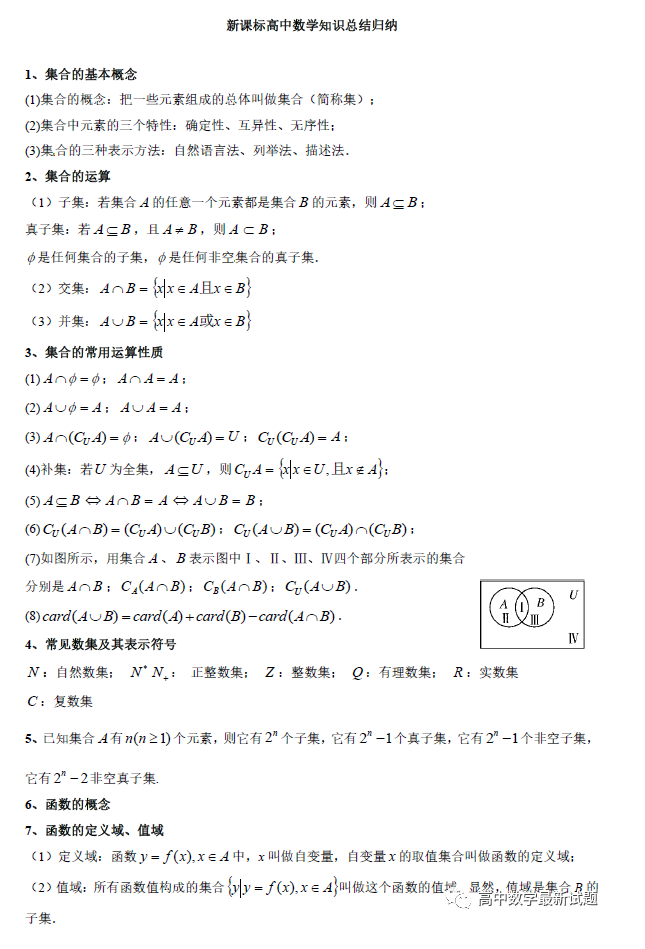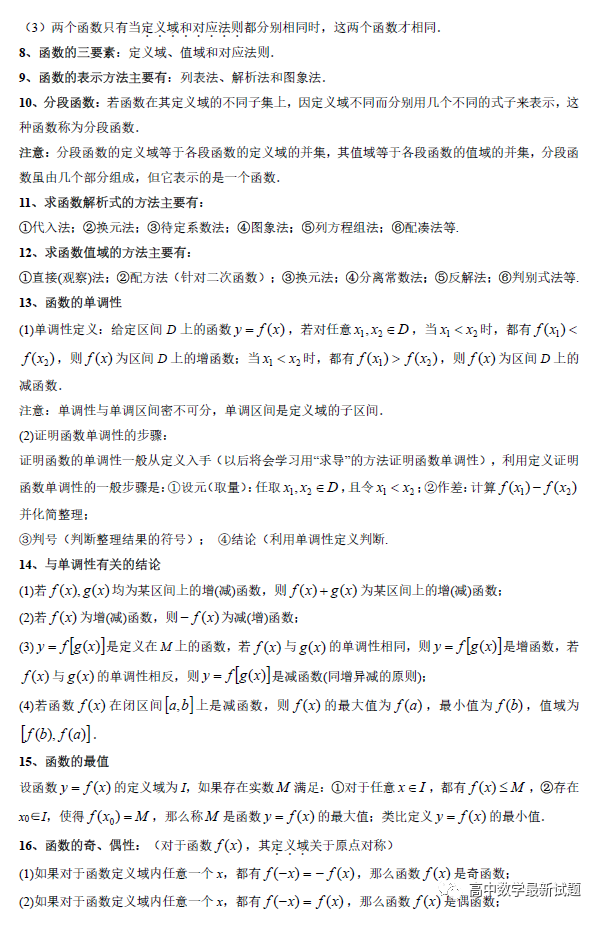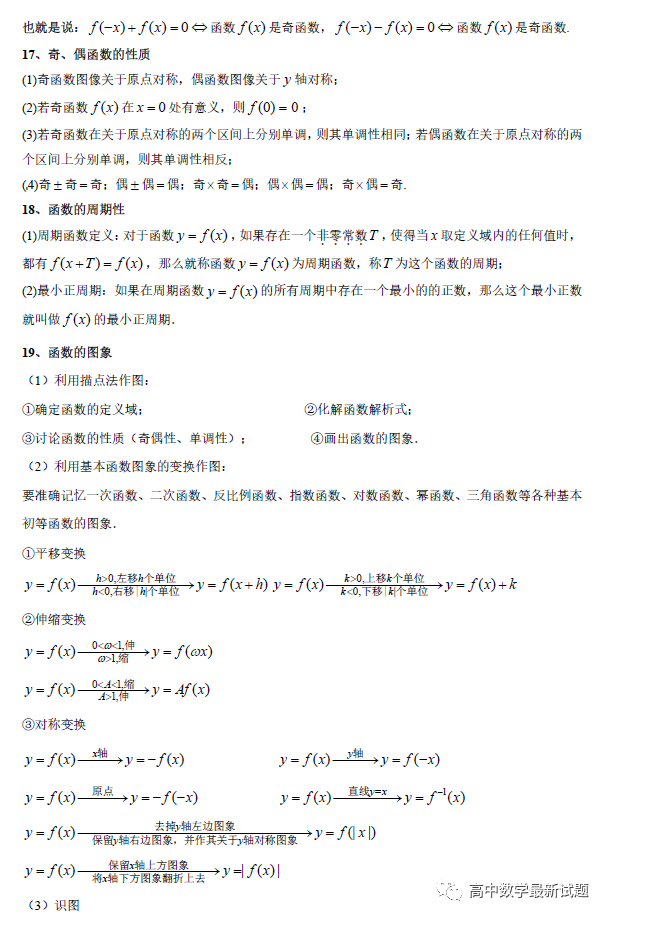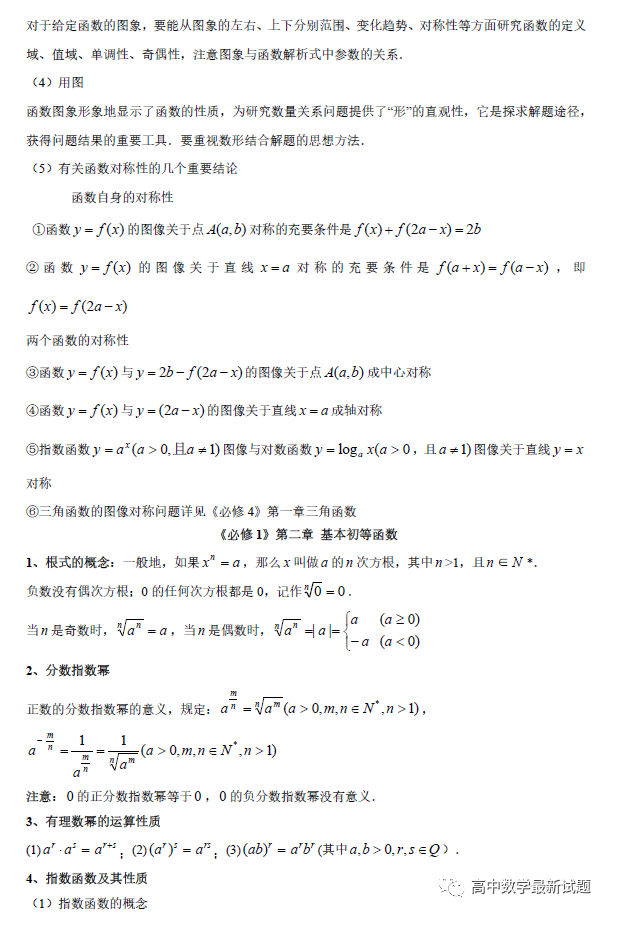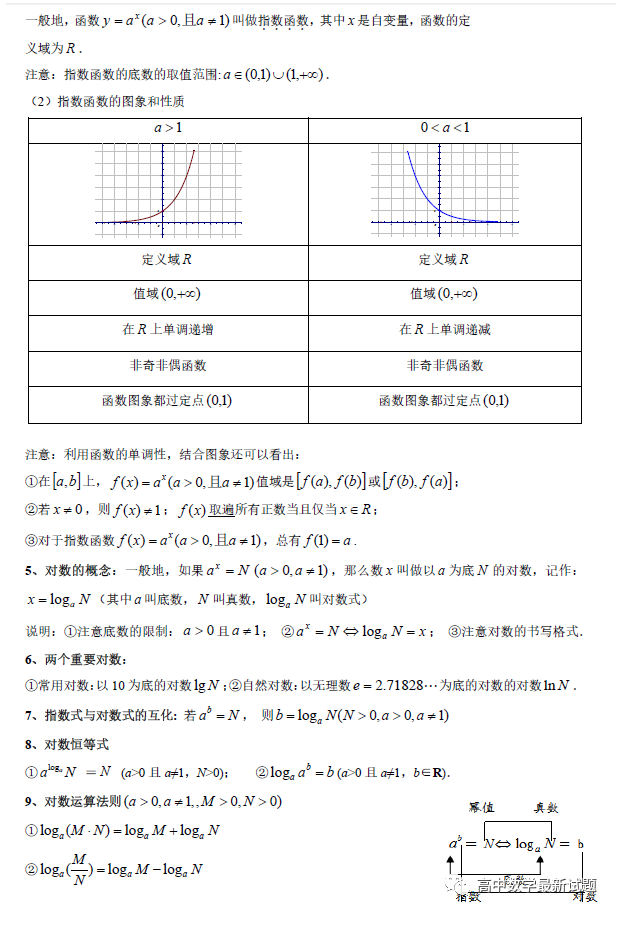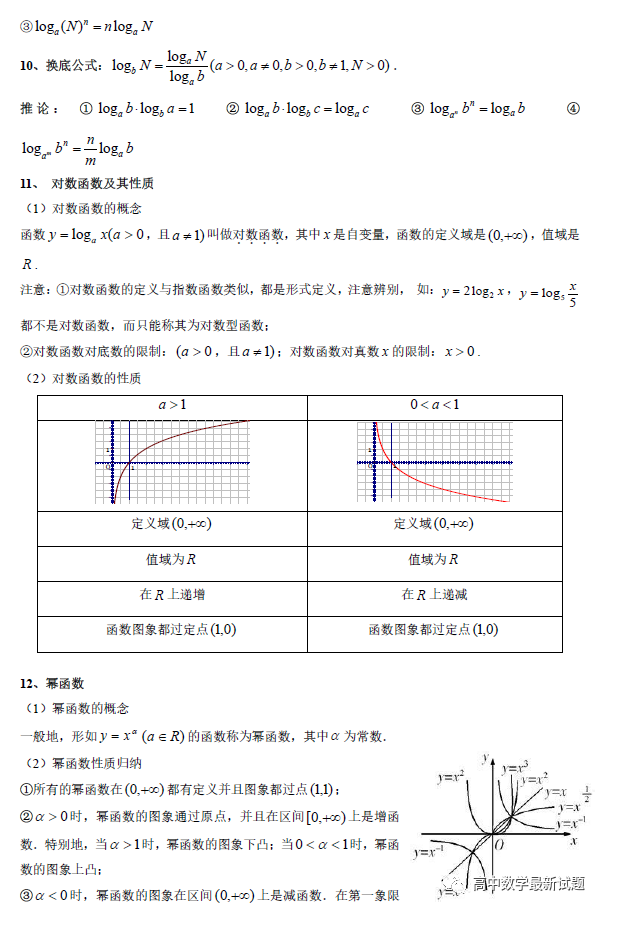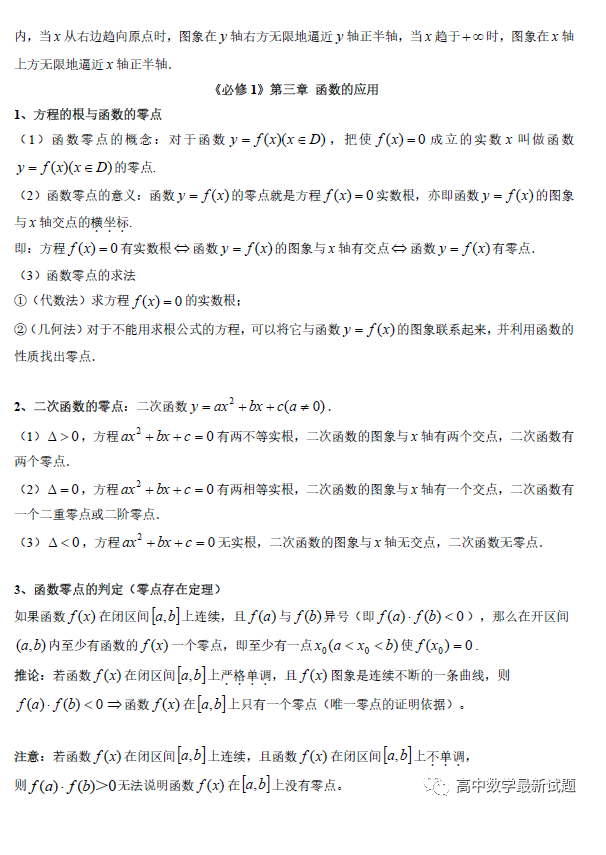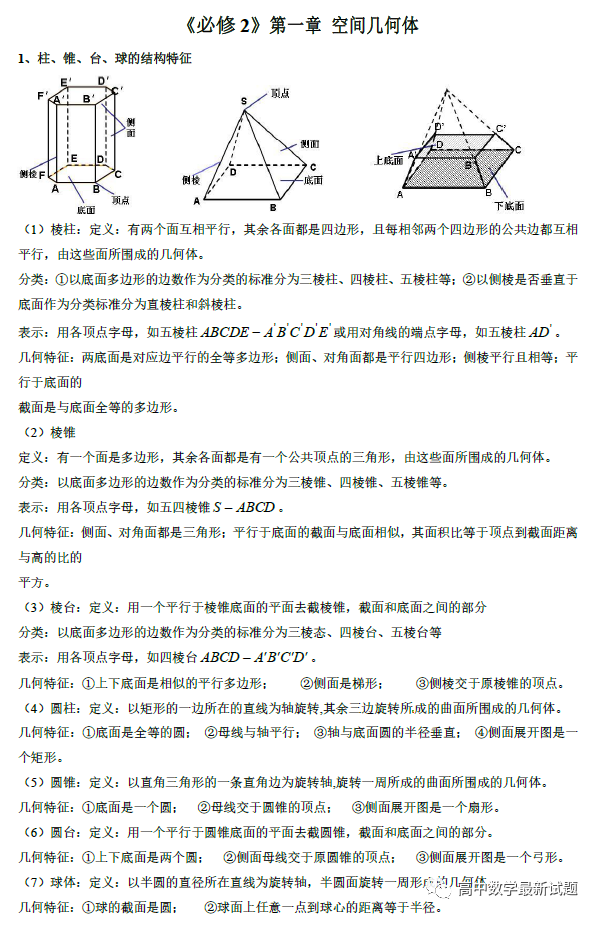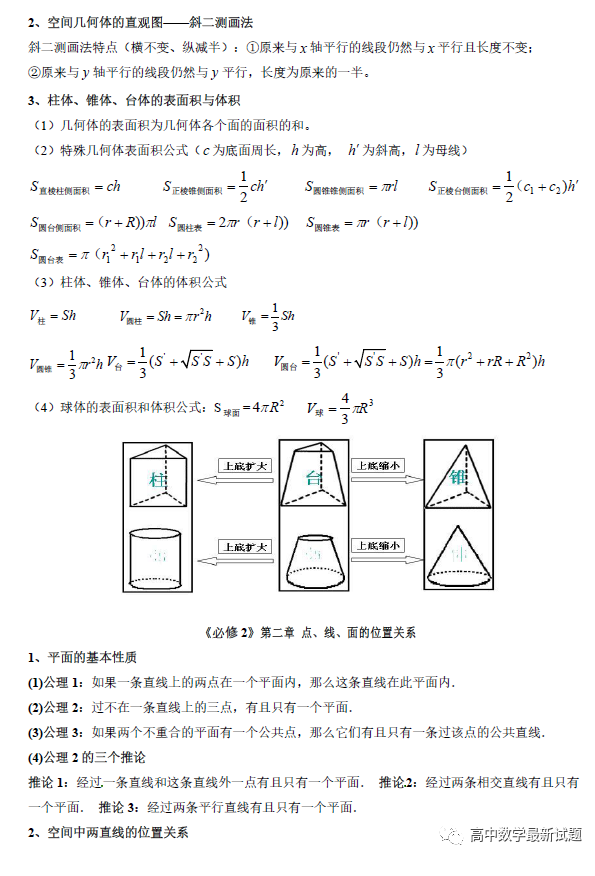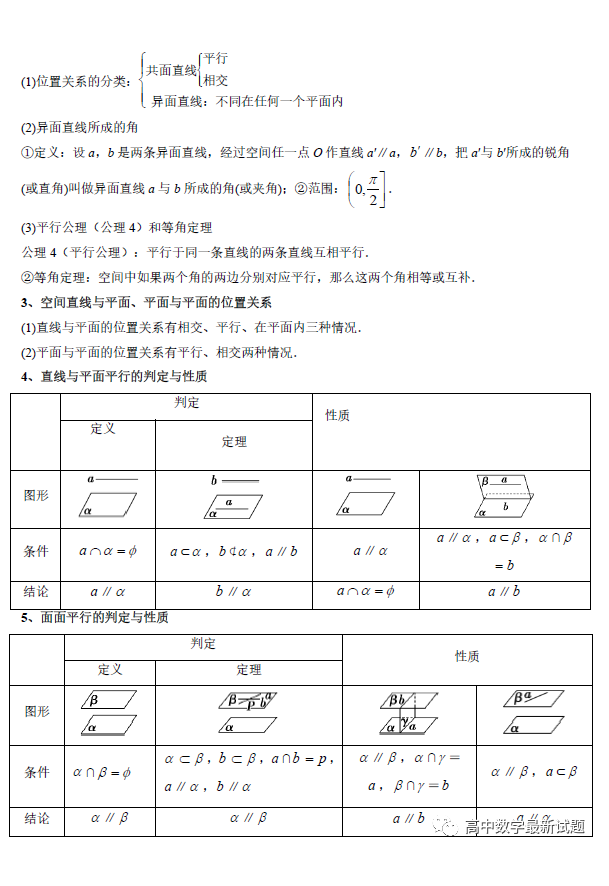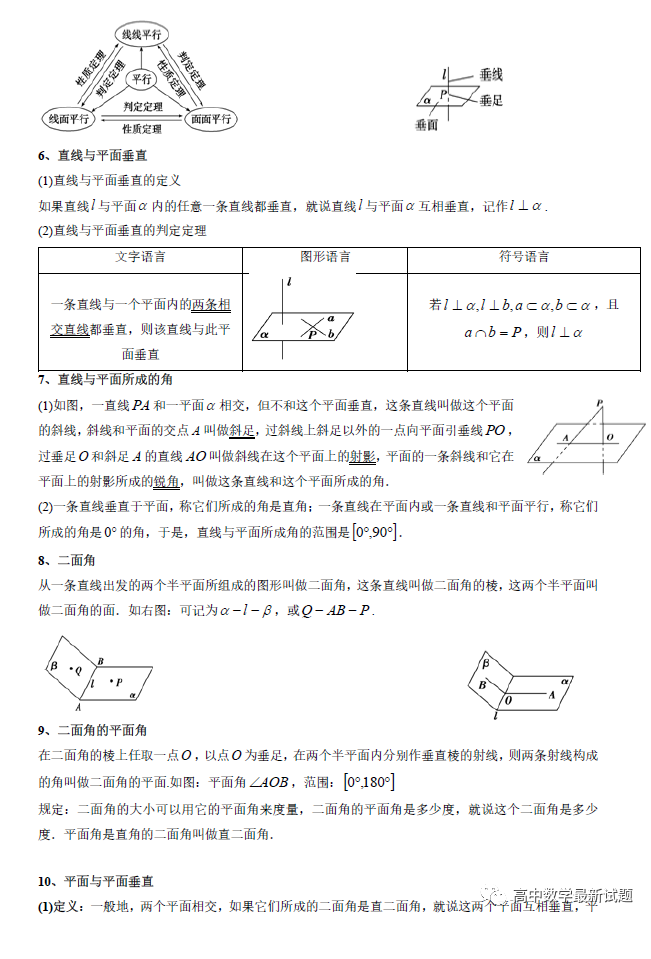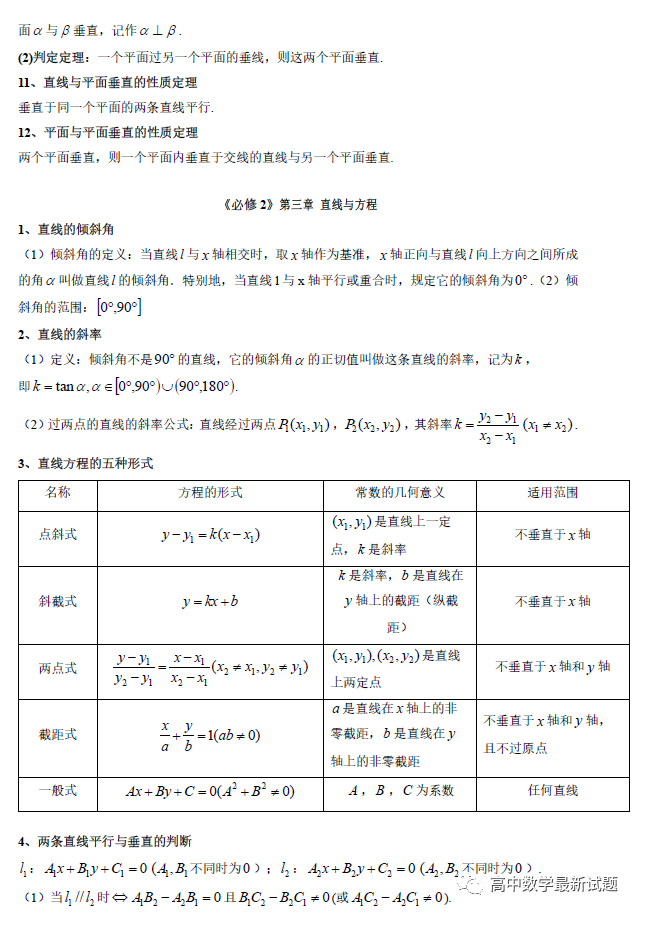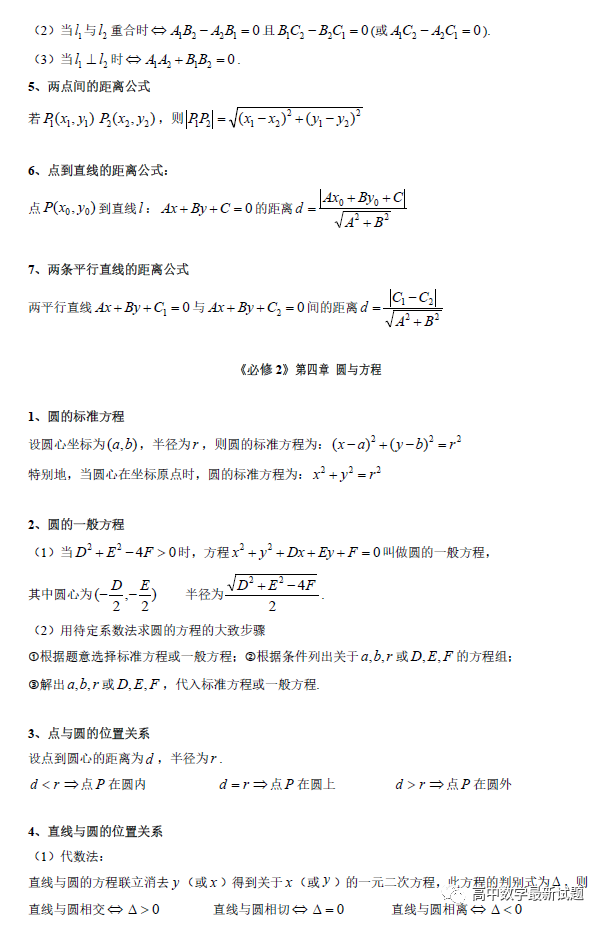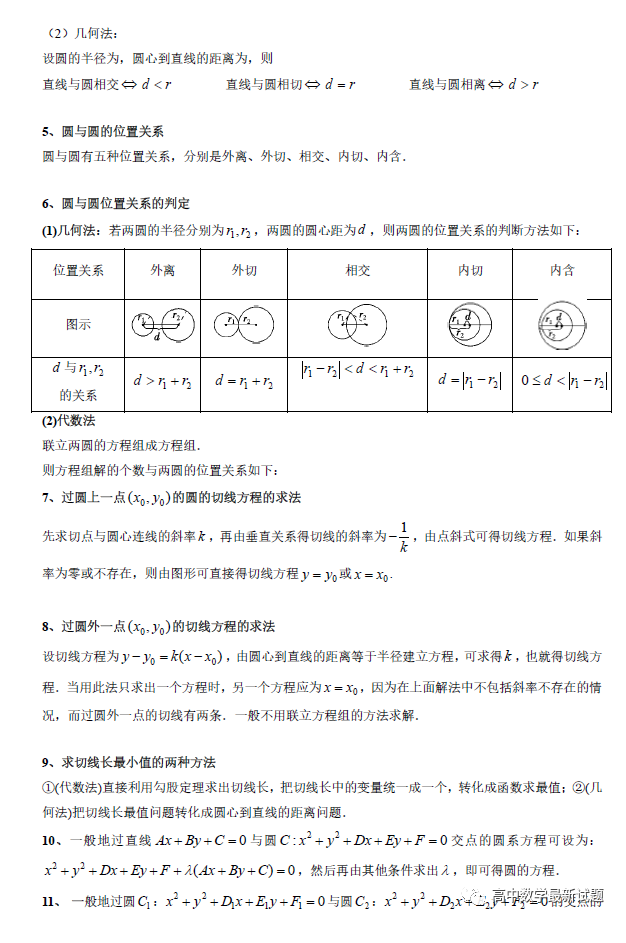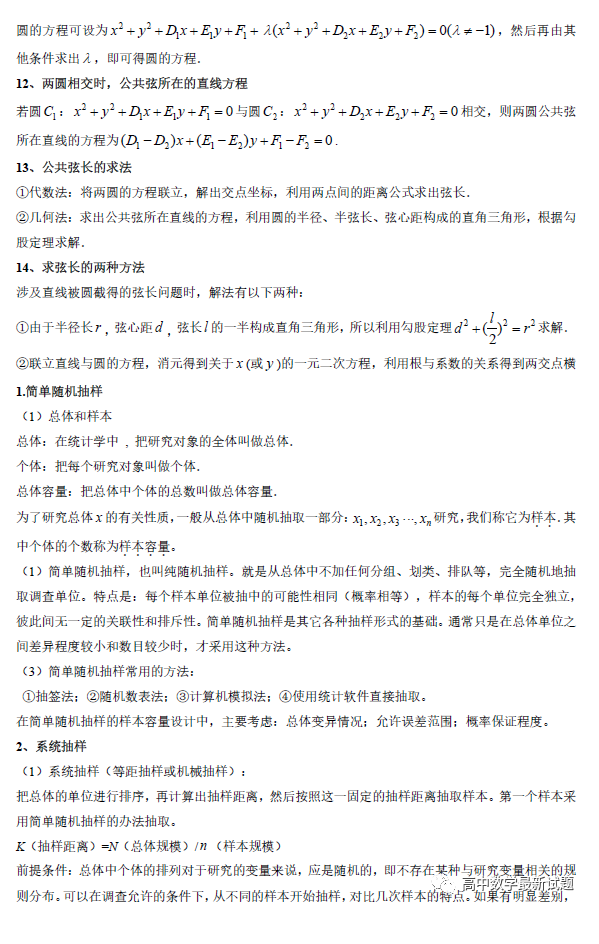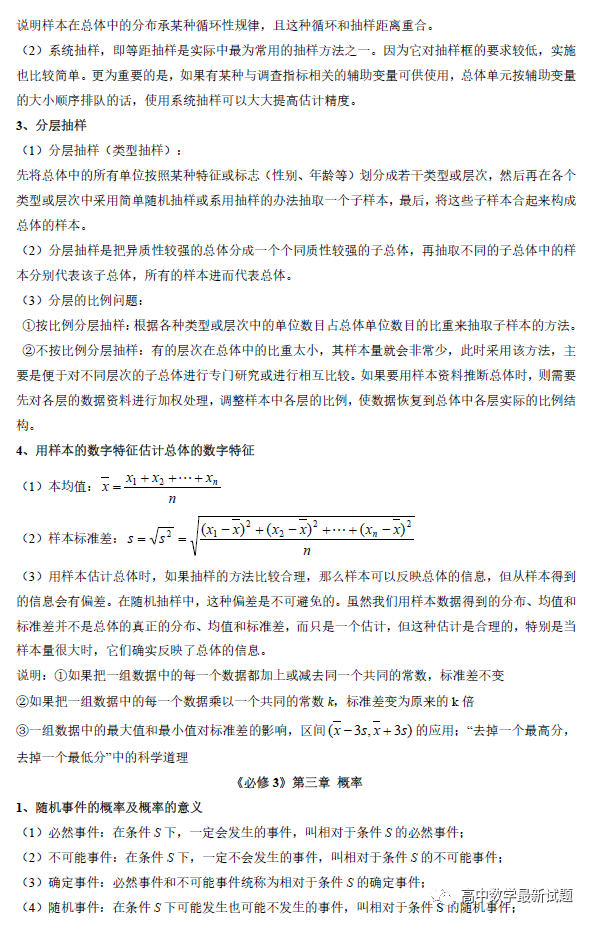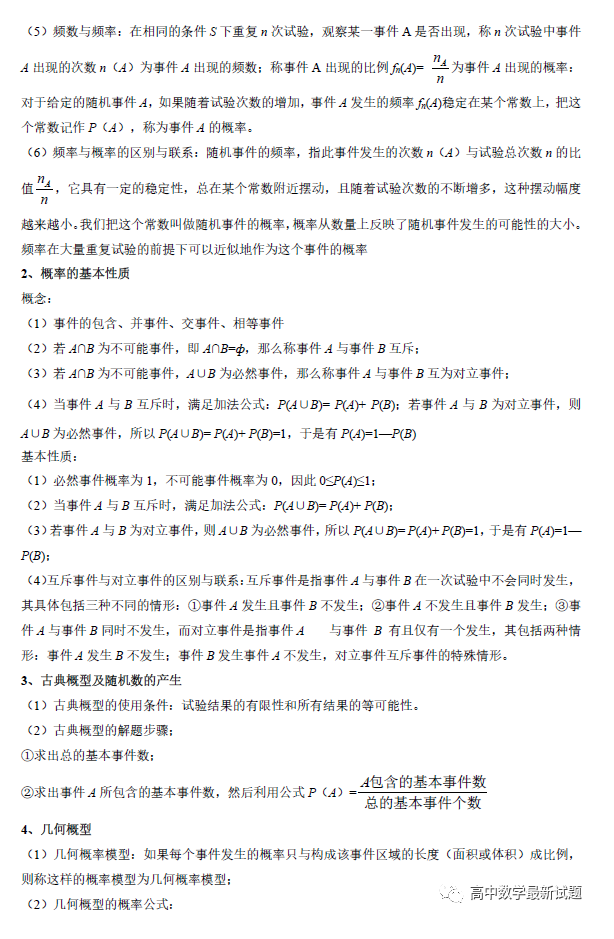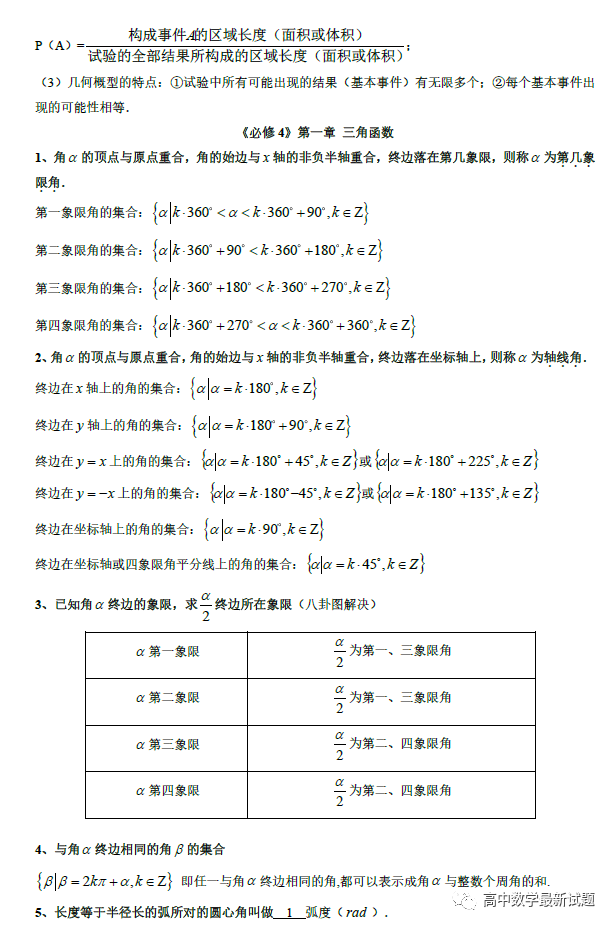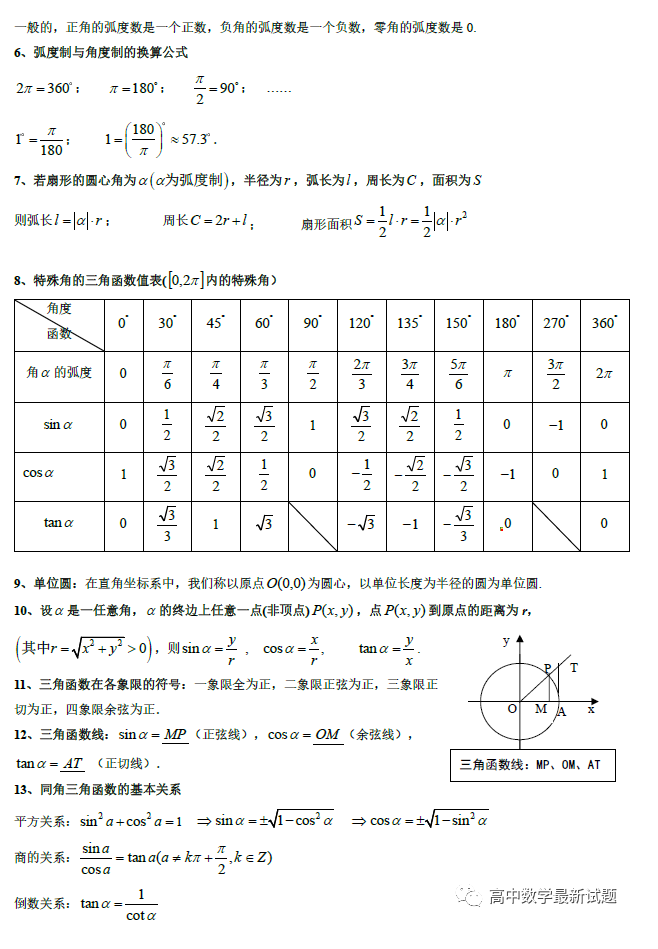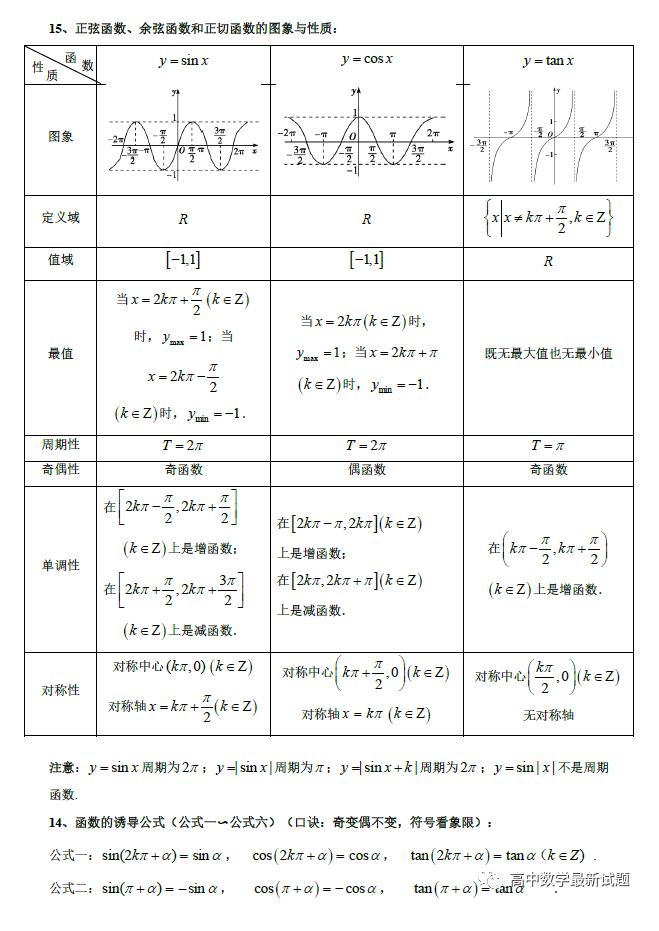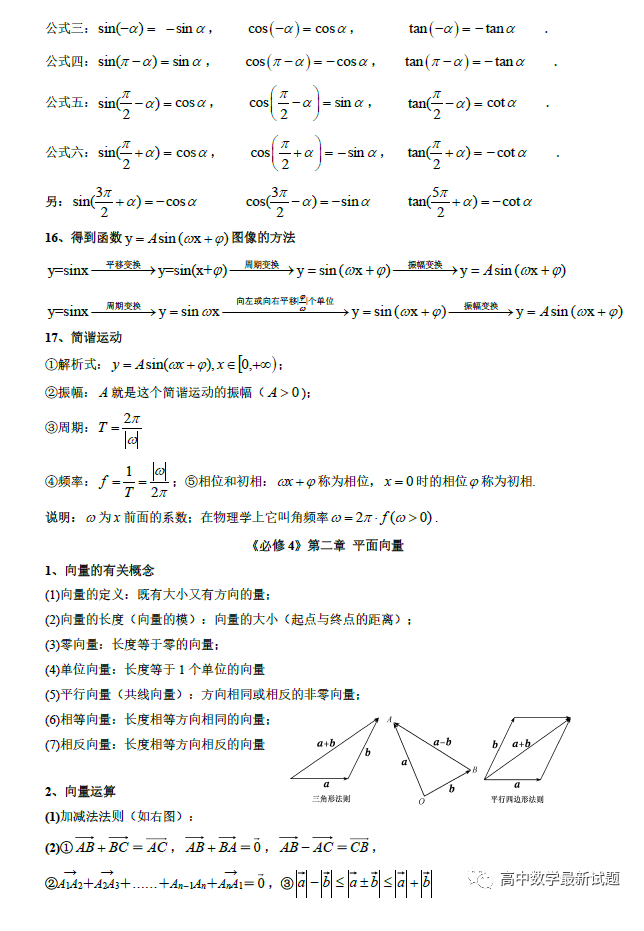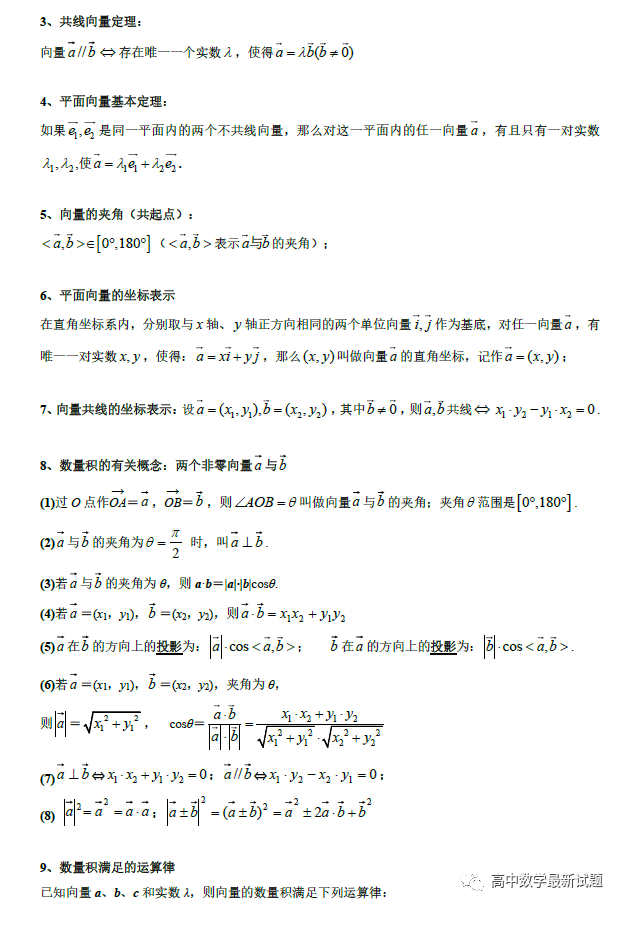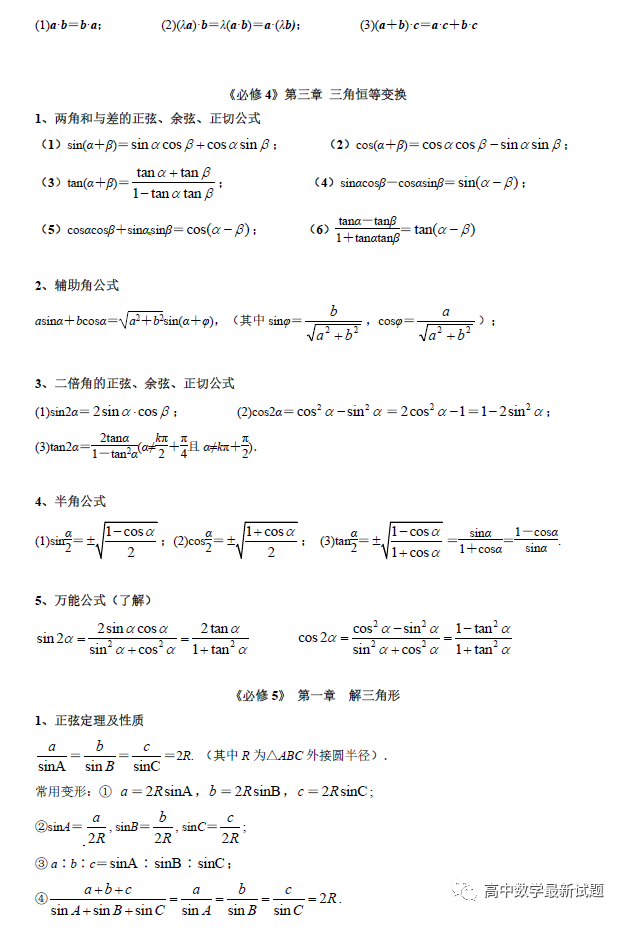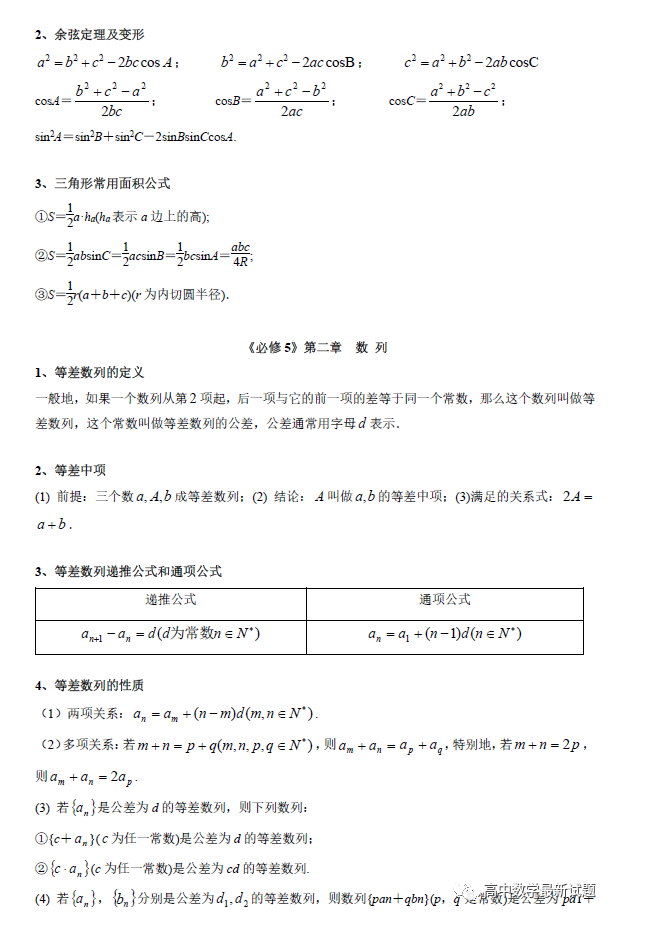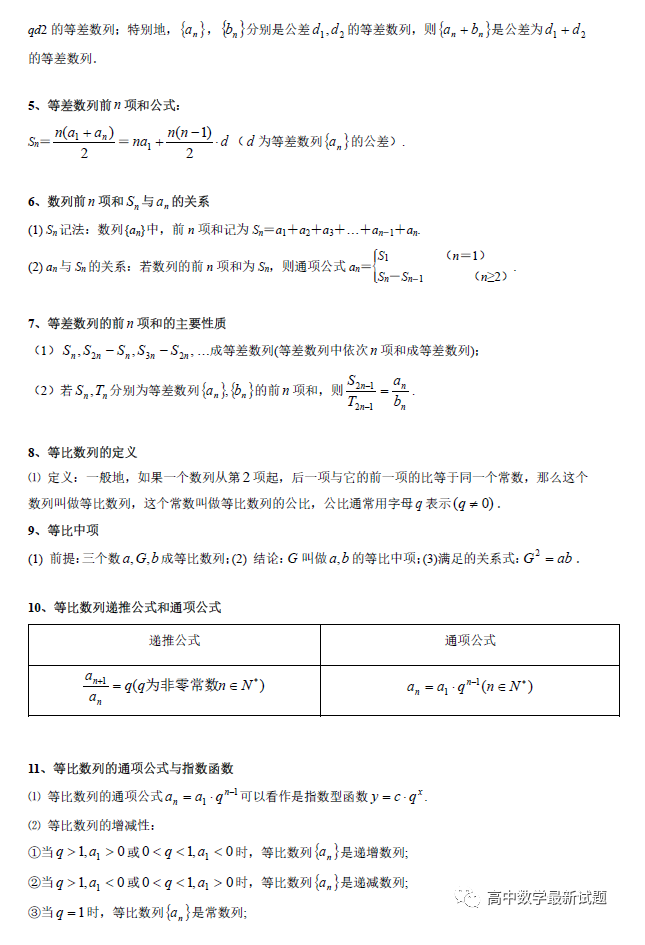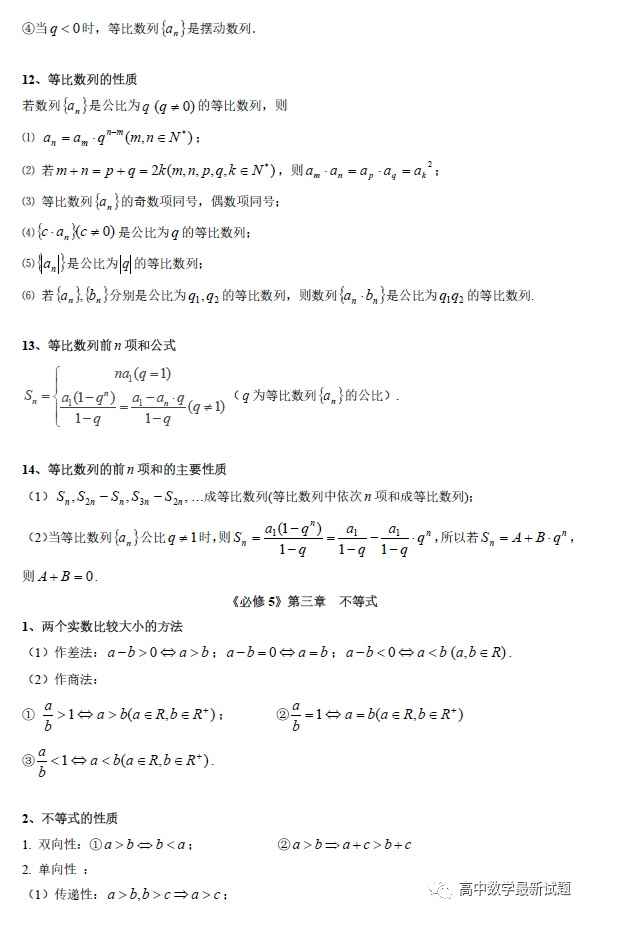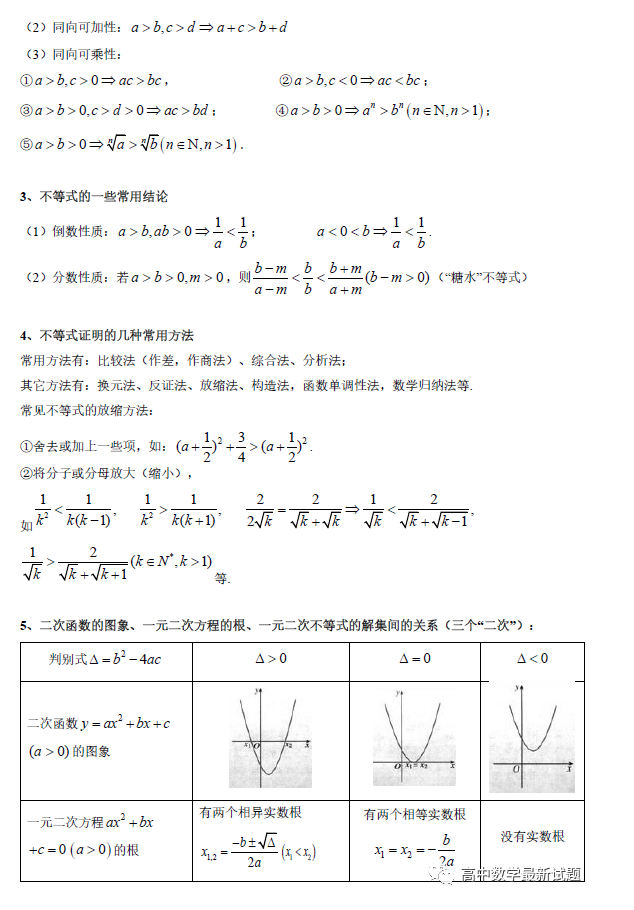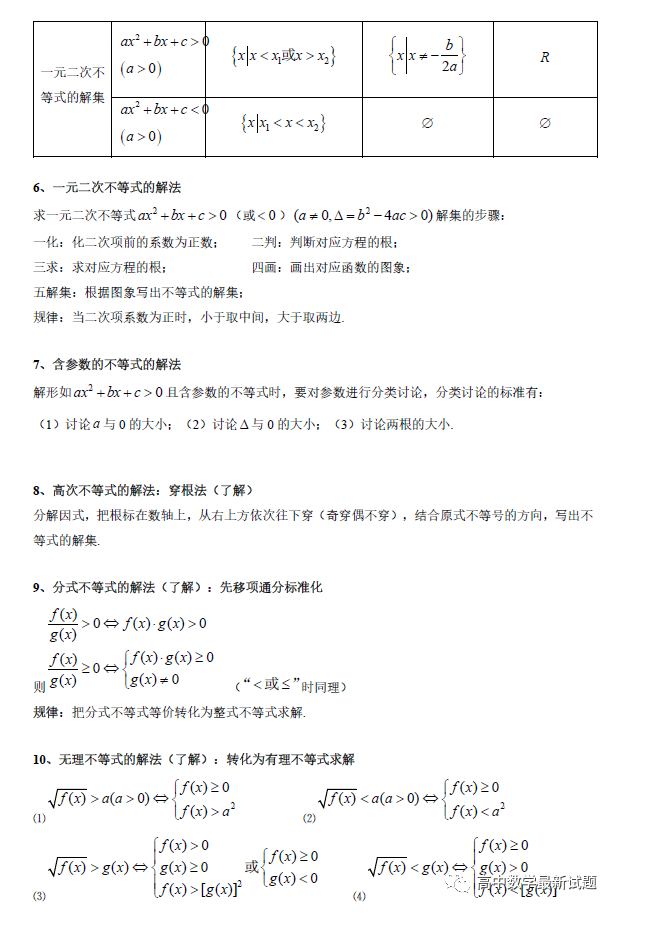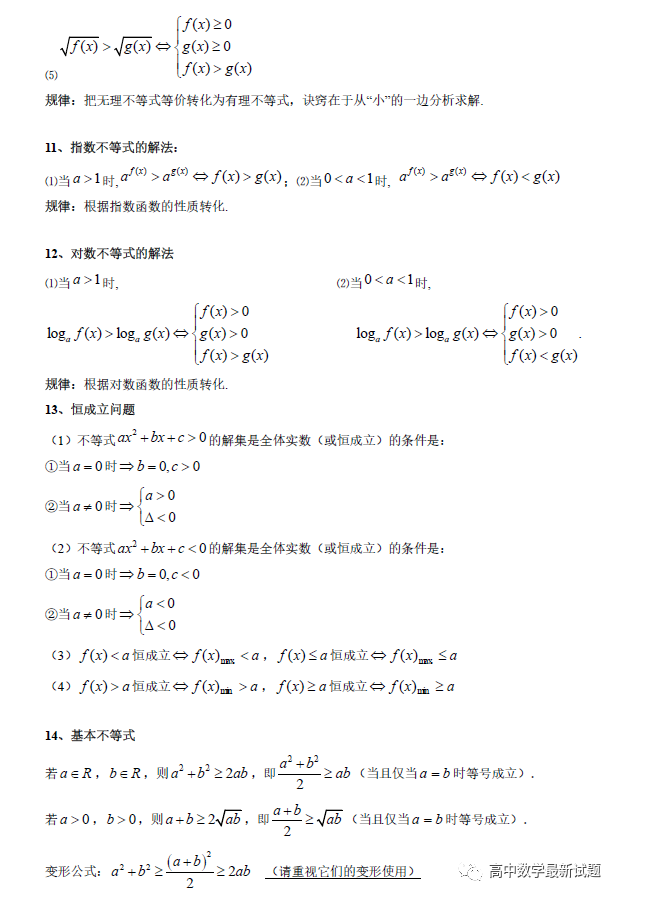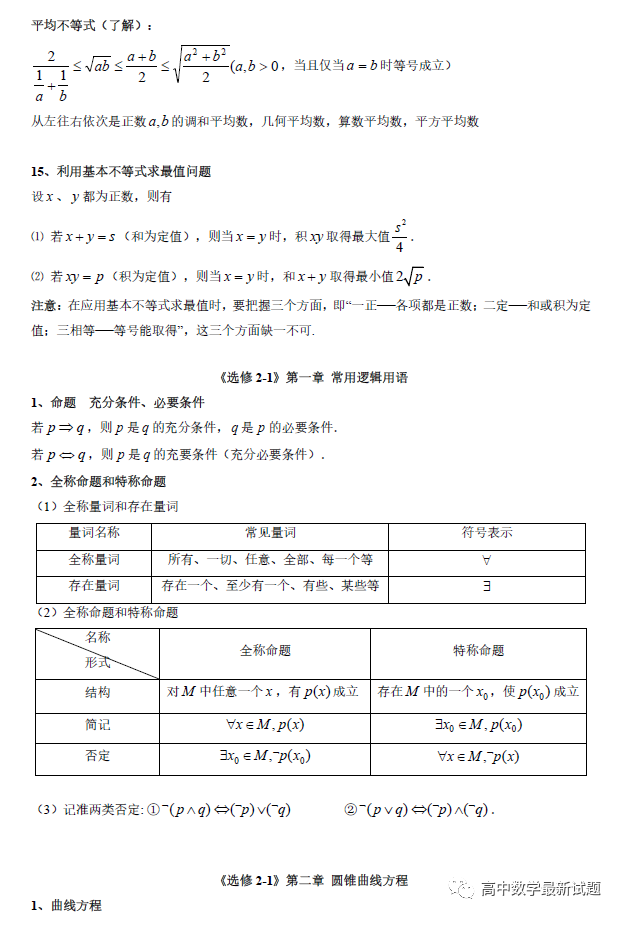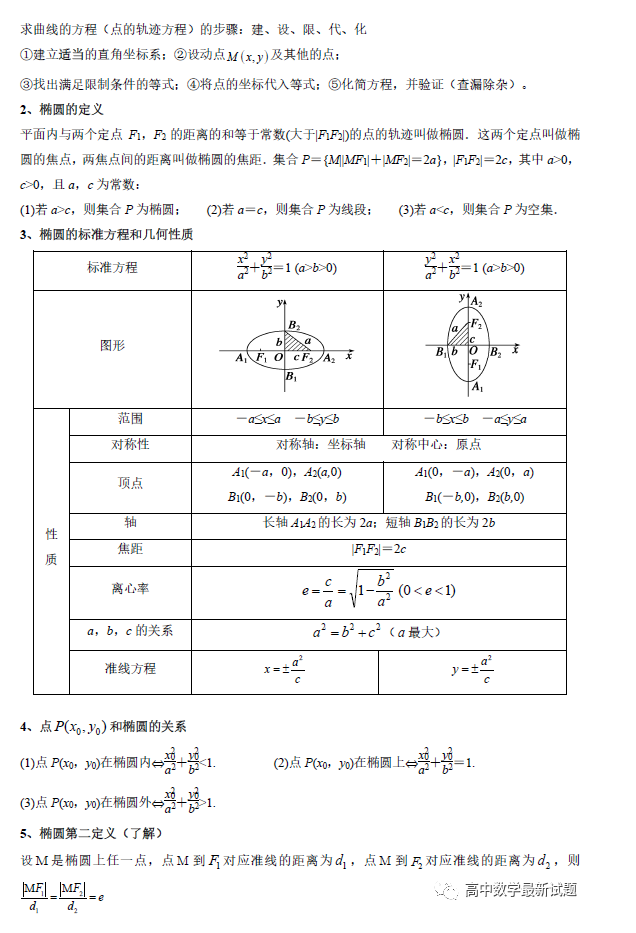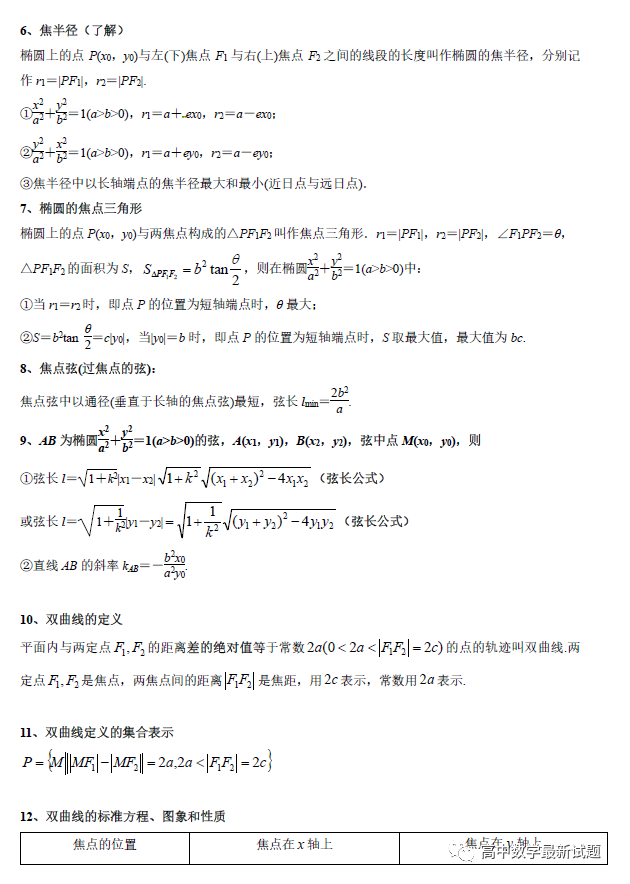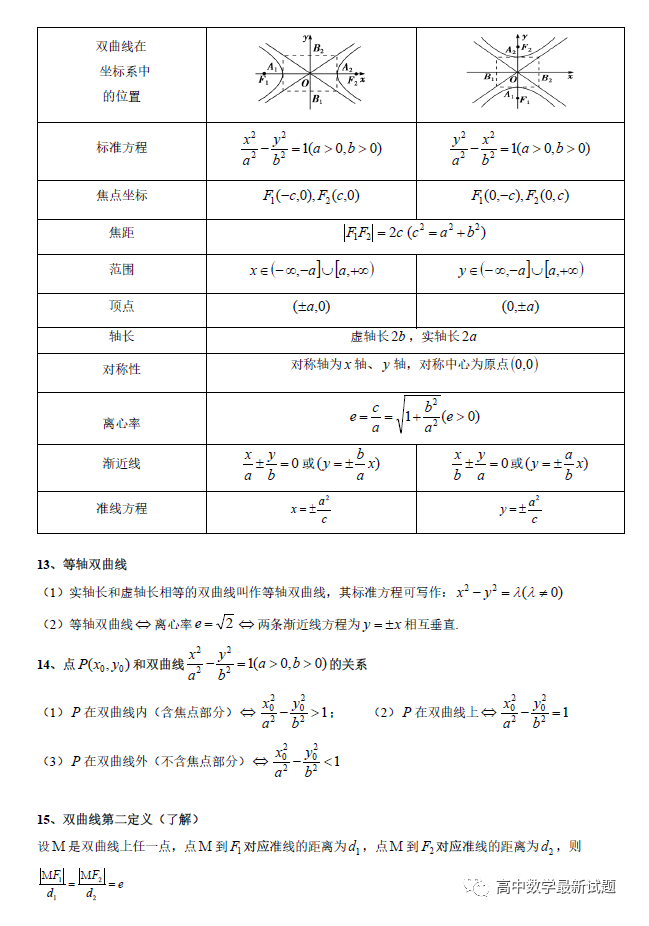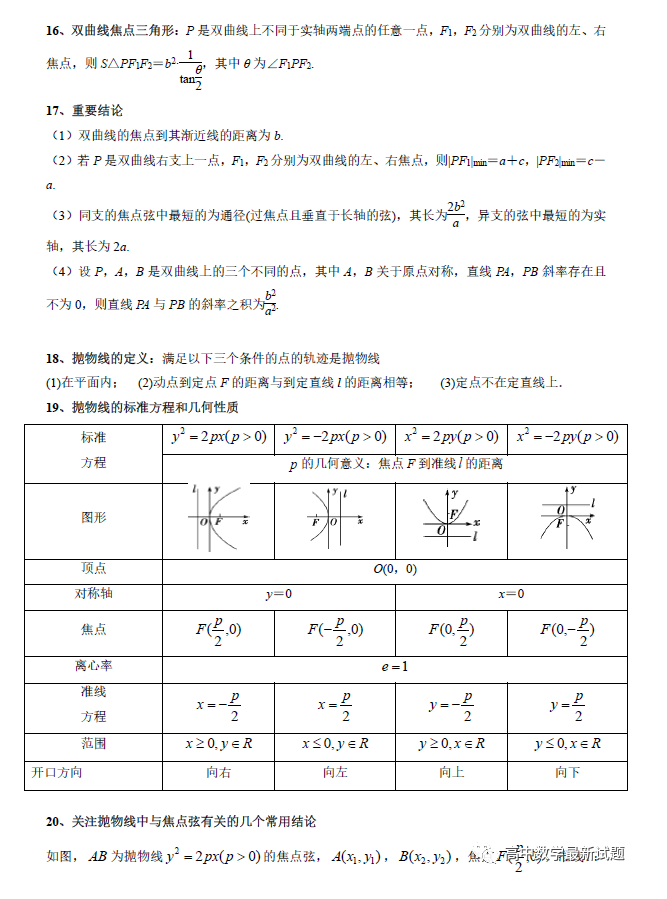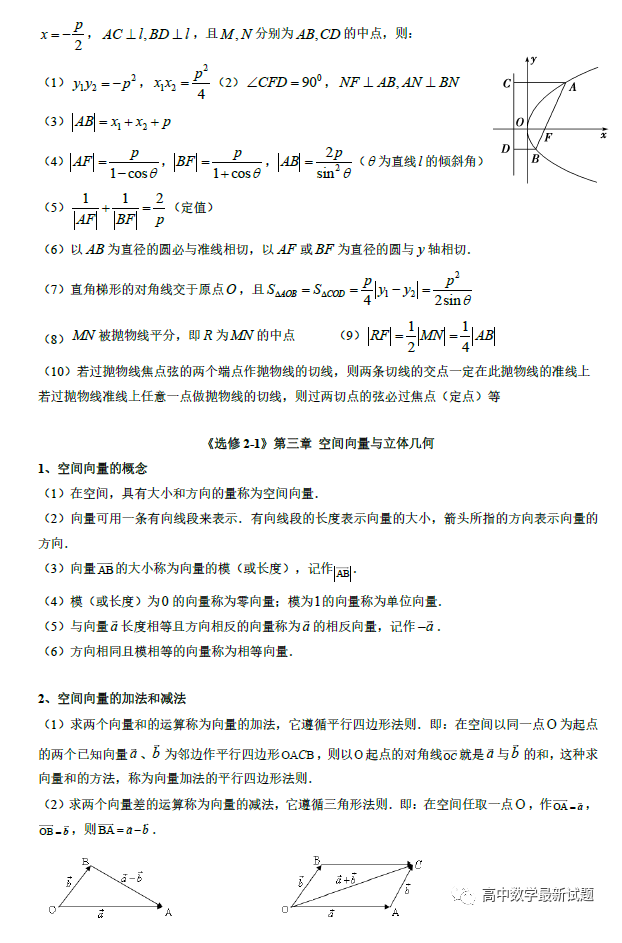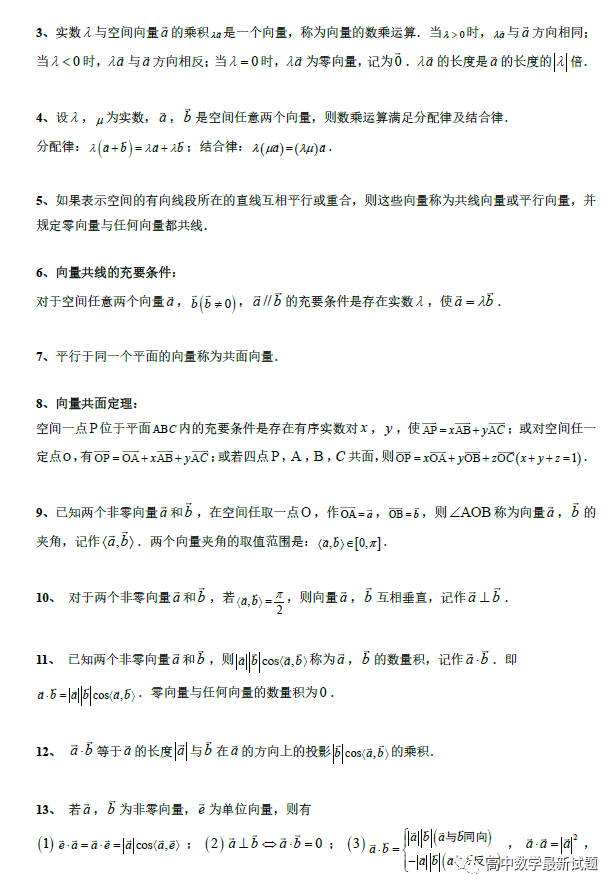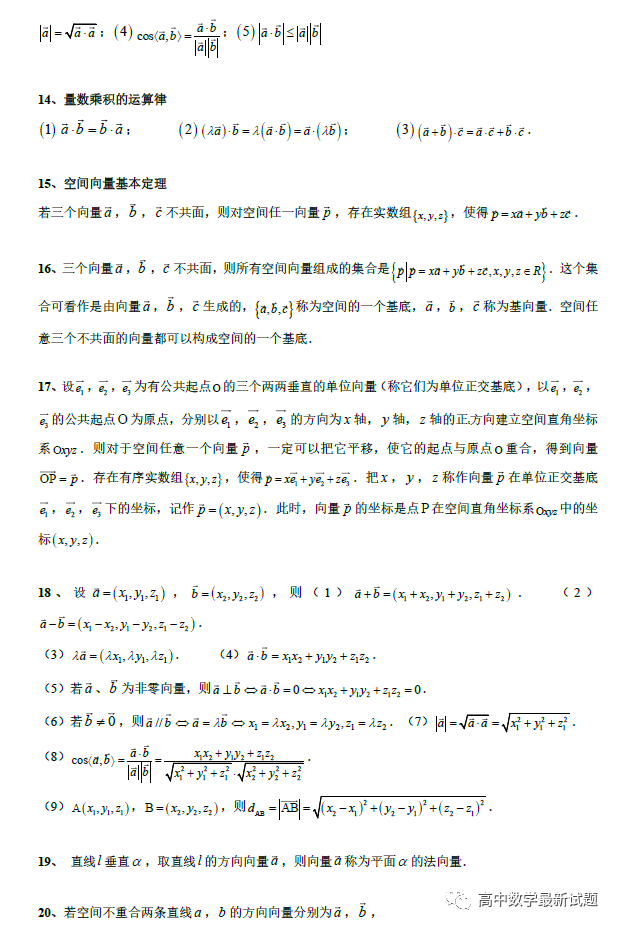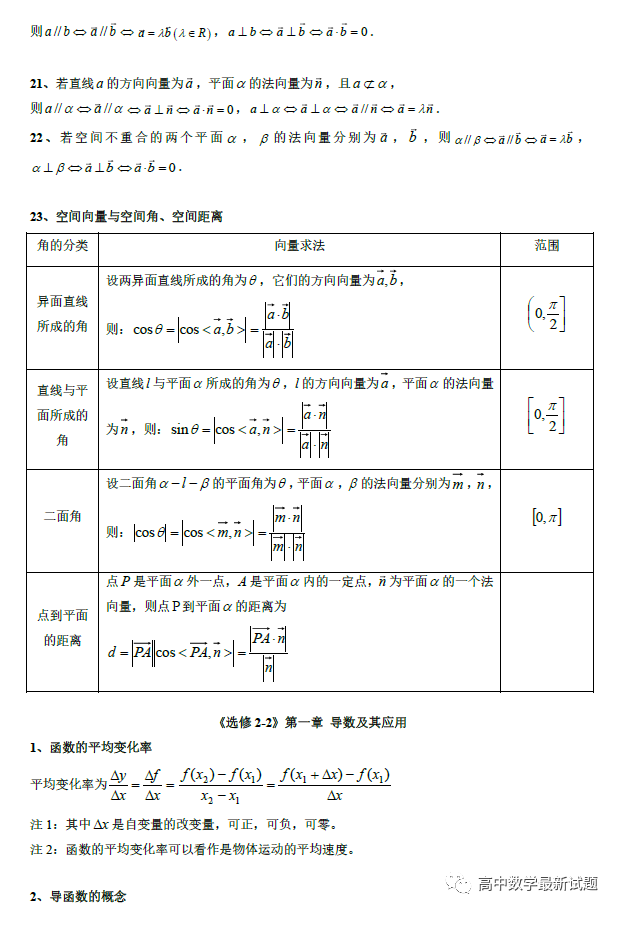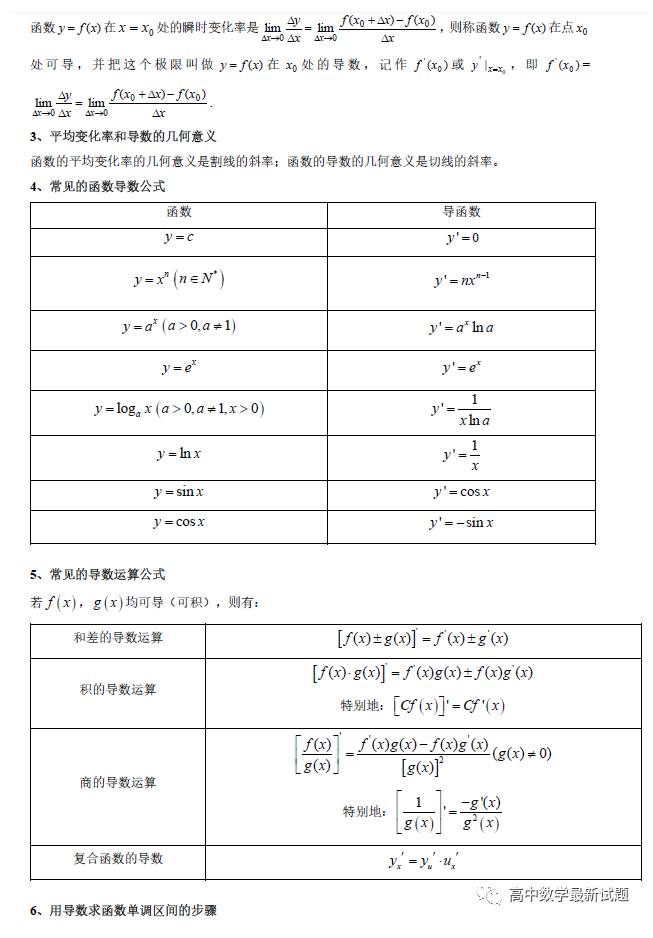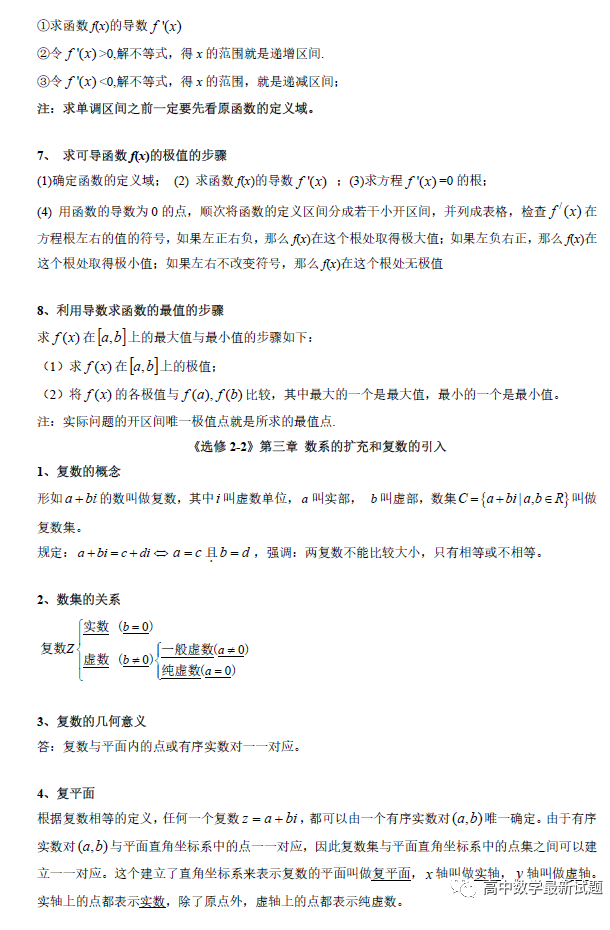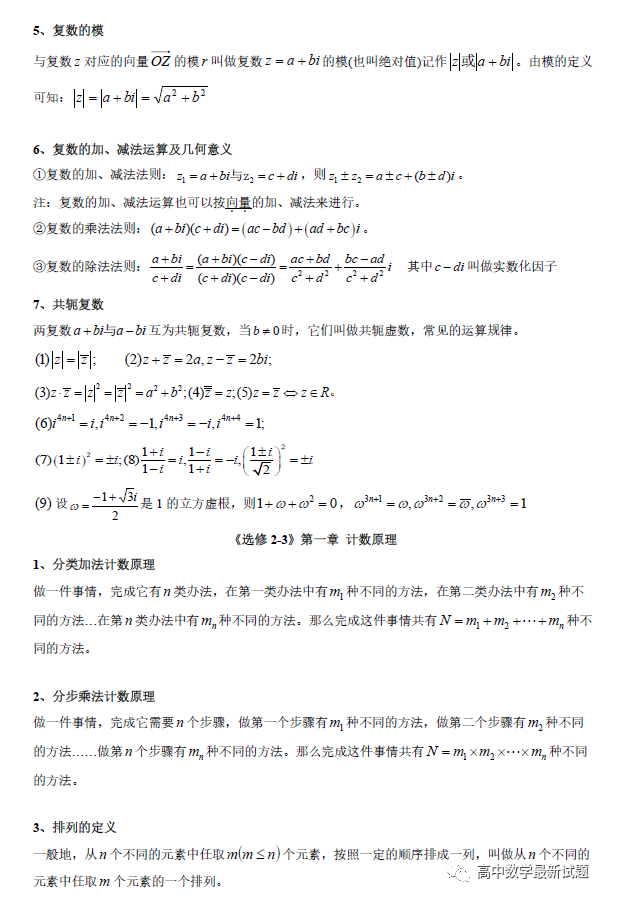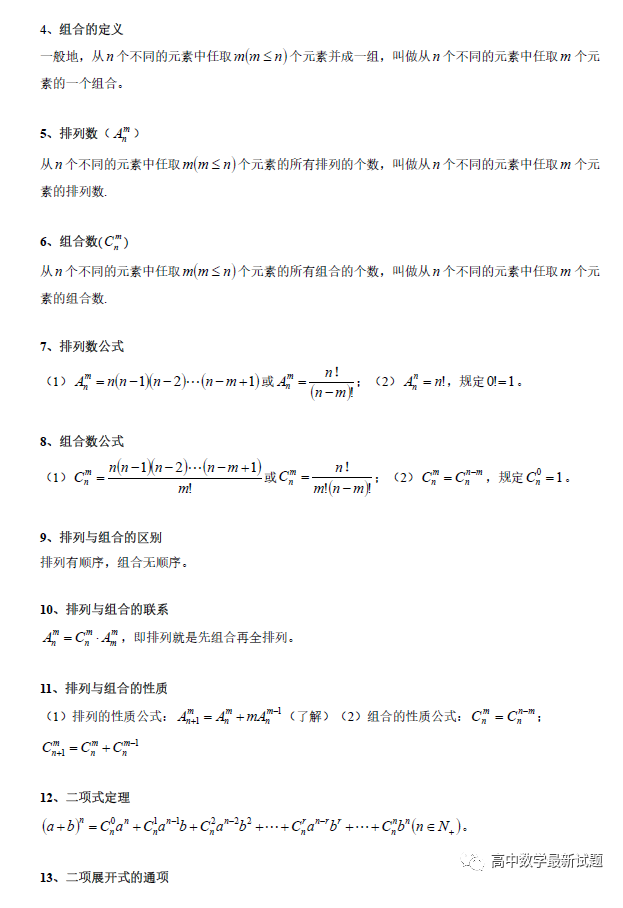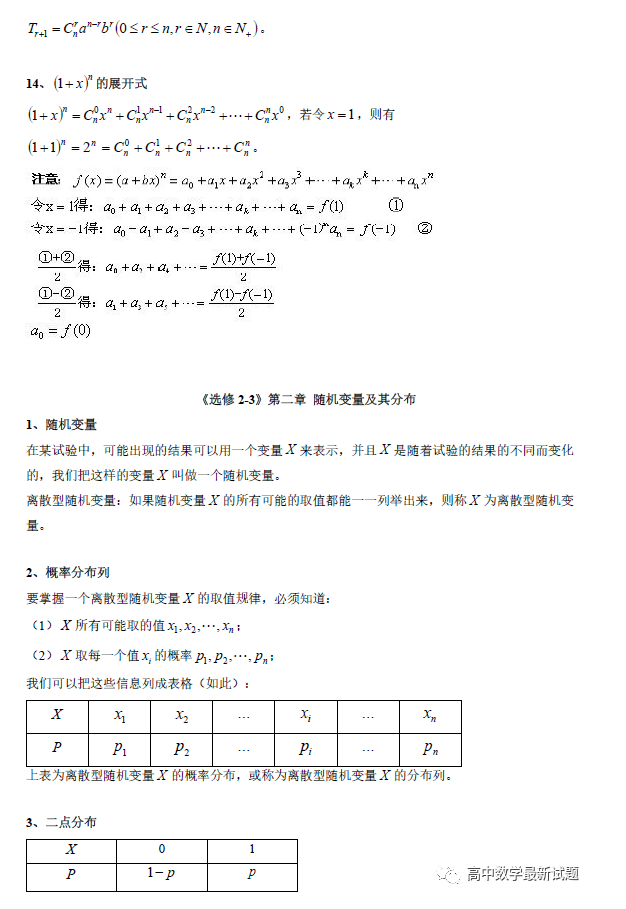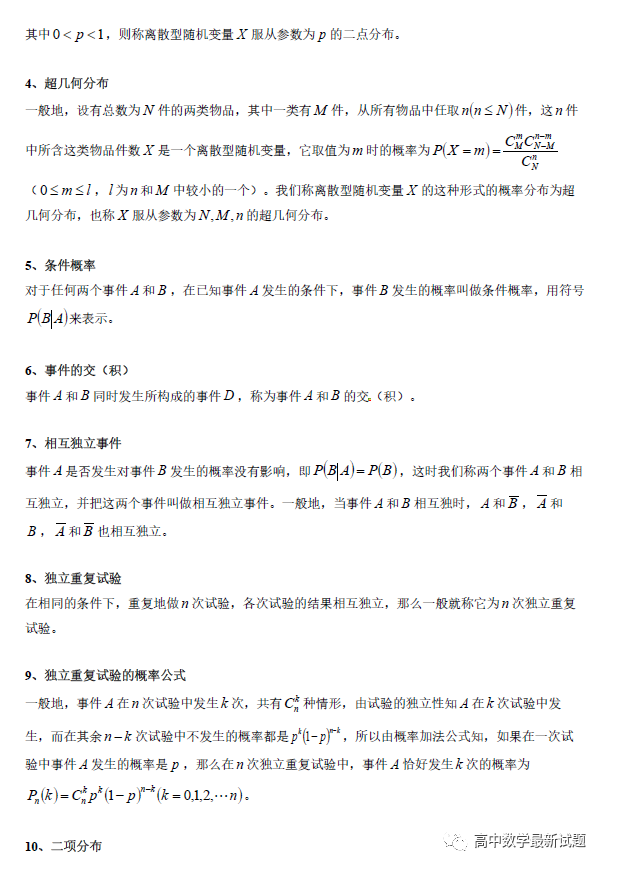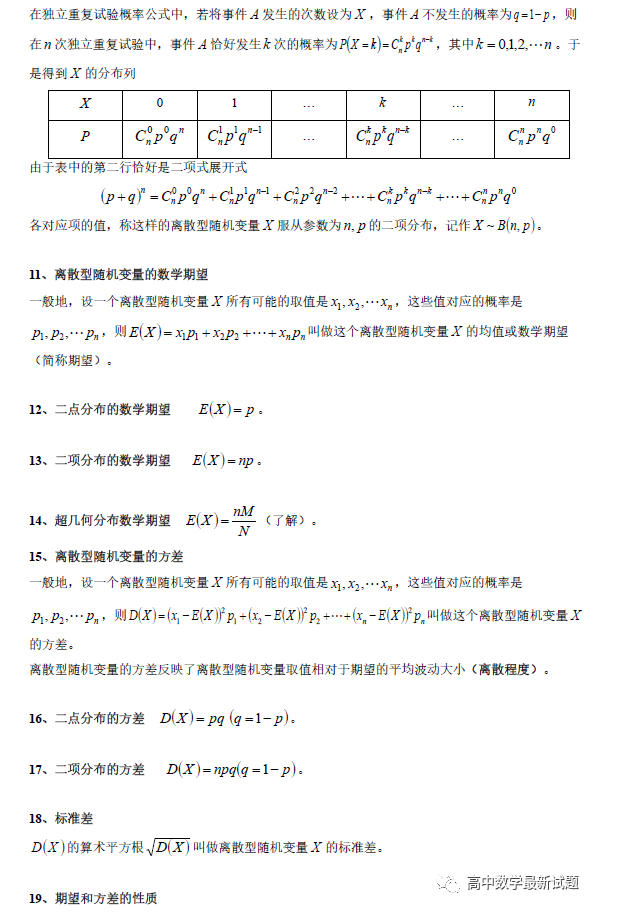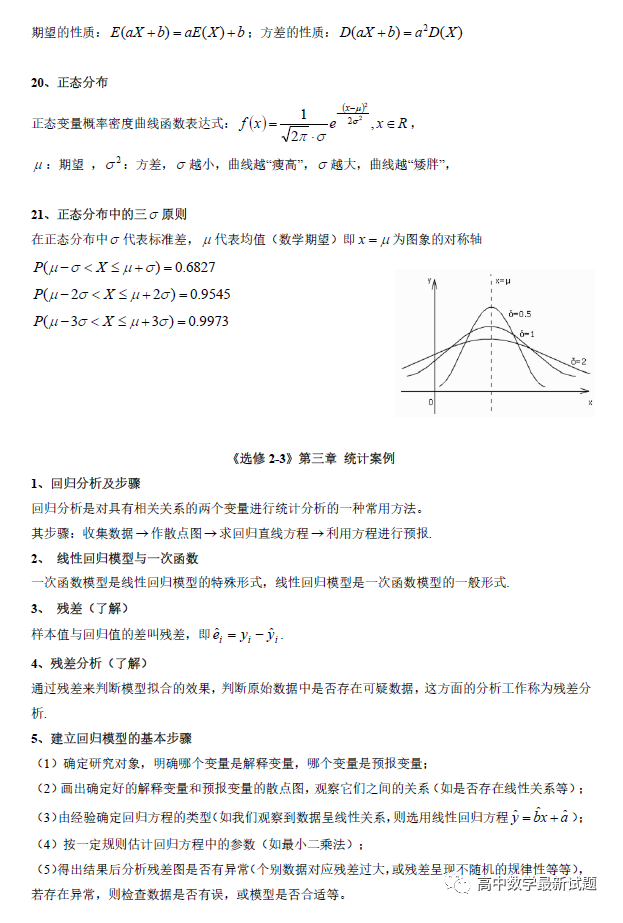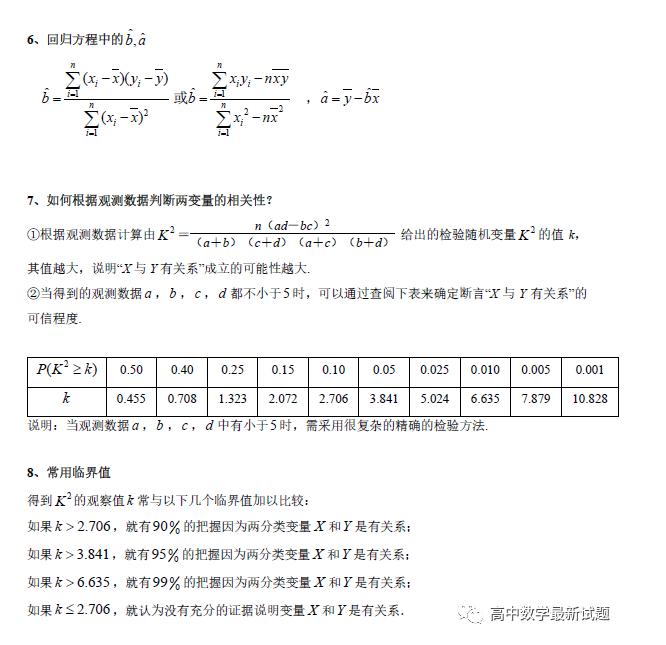### 二. 高考数学解题技巧及公式大全

1. 高考数学必考题型 之 函数与导数

2. 高考数学必考题型 之 几何

3. 高考数学必考题型 之 不等式

4. 高考数学必考题型 之 数列

(1)理解数列的概念，了解数列通项公式的意义了解递推公式是给出数列的一种方法，并能根据递推公式写出数列的前几项。

(2)理解等差数列的概念，掌握等差数列的通项公式与前n项和公式，并能解决简单的实际问题。

(3)理解等比数列的概念，掌握等比数列的通项公式与前n项和公式，井能解决简单的实际问题。

5. 必背公式

（1.）一元二次方程的解

-b+√(b2-4ac)/2a-b-√(b2-4ac)/2a

b2-4ac>0注：方程有两个不相等的个实根

b2-4ac<0注：方程有共轭复数根

（2）立体图形及平面图形的公式

（3）图形周长、面积、体积公式

sin(A+B)=sinAcosB+cosAsinB sin(A-B)=sinAcosB-sinBcosA

cos(A+B)=cosAcosB-sinAsinB cos(A-B)=cosAcosB+sinAsinB

tan(A+B)=(tanA+tanB)/(1-tanAtanB) tan(A-B)=(tanA-tanB)/(1+tanAtanB)

ctg(A+B)=(ctgActgB-1)/(ctgB+ctgA) ctg(A-B)=(ctgActgB+1)/(ctgB-ctgA)

tan2A=2tanA/(1-tan2A) ctg2A=(ctg2A-1)/2ctga

cos2a=cos2a-sin2a=2cos2a-1=1-2sin2a

sin(A/2)=√((1-cosA)/2) sin(A/2)=-√((1-cosA)/2)

cos(A/2)=√((1+cosA)/2) cos(A/2)=-√((1+cosA)/2)

tan(A/2)=√((1-cosA)/((1+cosA)) tan(A/2)=-√((1-cosA)/((1+cosA))

ctg(A/2)=√((1+cosA)/((1-cosA)) ctg(A/2)=-√((1+cosA)/((1-cosA))

2sinAcosB=sin(A+B)+sin(A-B) 2cosAsinB=sin(A+B)-sin(A-B)

2cosAcosB=cos(A+B)-sin(A-B) -2sinAsinB=cos(A+B)-cos(A-B)

sinA+sinB=2sin((A+B)/2)cos((A-B)/2 cosA+cosB=2cos((A+B)/2)sin((A-B)/2)

tanA+tanB=sin(A+B)/cosAcosB tanA-tanB=sin(A-B)/cosAcosB

ctgA+ctgBsin(A+B)/sinAsinB -ctgA+ctgBsin(A+B)/sinAsinB

9

10

### 手机验证